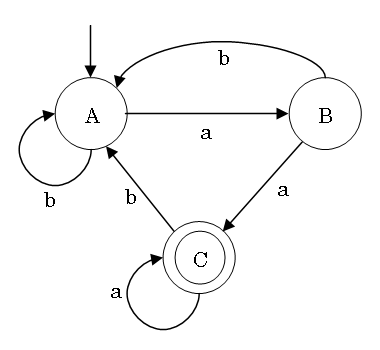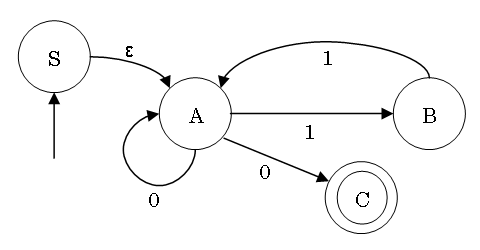# Finite State Automata and Linear Grammars

(有限オートマトンと線形文法)

## Language Theory and Compilers

### 3rd lecture, April 21, 2017

http://www.sw.it.aoyama.ac.jp/2017/CP1/lecture3.html

### Martin J. Dürst© 2005-17 Martin J. Dürst 青山学院大学

# Today's Schedule

• Leftovers (grammar types) and homework from last lecture
• Finite state automata
• Linear grammars
• Conversions

# Cygwin Download and Installation

(no need to submit, but bring your note PC with you if you have problems)
On your note PC, install cygwin (detailled instructions with screenshots).
Make sure you select/install all of gcc, flex, bison, diff, make and m4.

# Checking `flex`, `bison`, `gcc`,... Installation

To check your installation of the various programs, start up a Cygwin Terminal session, and use the following commands to check the version of each software:

• `flex -V` (`V` is upper case)
• `bison -V` (`V` is upper case)
• `gcc -v` (`v` is lower case)
• `diff -v` (`v` is lower case)
• `make -v` (`v` is lower case)
• `m4 --version`

# Summary of Last Lecture

 grammar type lanugage type automaton phrase structure grammar (psg) 0 phrase structure language Turing machine context-sensitive grammar (csg) 1 context-sensitive language linear-bounded automaton context-free grammar (cfg) 2 context-free language push-down automaton regular grammar (rg) 3 regular language finite state automaton

Regular languages are used for lexical analysis.

# Plan for this Lecture

• Finite state automata (FSA)
• Deterministic finite automaton (DFA)
• Non-deterministic finite automaton (NFA)
• Regular grammar
• Left linear grammar
• Right linear grammar
• [Regular expression]

These all are equivalent, and define/accept regular languages

# Finite State Automaton Example

(automaton (αὐτόματον) is Greek; plural: automata)

Finite state automata are often represented with a state transition diagramArrow from outside: initial state
Circles: states
Double circles: accepting state(s)
Arrows with labels: transitions

# Workings of a Finite State Automaton

• Start with initial state
• Repeatedly read one symbol of the input word,
and transition to the next state along the arrow with the corresponding label
• If the automaton is in an accepting state at the end of the word,
then the word is accepted
• If the automaton is not in an accepting state at the end of the word,
or if there is no label with the right symbol, then the word is not accepted
• The number of states is finite (i.e. there is only limited memory)

# Examples of Finite State Automata

• Accepting only a word with a single specific symbol
• Accepting words where the number of symbols is odd, or even, or when divided by 3, the reminder is 2,...
• Accepting words with a fixed sequence of symbols at the start
• Accepting words with a fixed sequence of symbols at the end
• Accepting words with a fixed sequence of symbols somewhere in the middle
• Accepting words meeting more than one condition, at the same time or one after the other, or one of more than one conditions

# State Transition Tables

Finite state automata can also be represented with state transition tables.

The state transition table for our example automaton is:

a b B A C A C A

Leftmost column: state
Top row: input symbol
→: start state (first state if not otherwise indicated)
*: accepting state(s)
Table contents: state after transition

# Formal Definition of FSAs

• A finite set of states Q (circles in diagram; leftmost column in table)
• A finite set of input symbols Σ (arrow labels in diagram; top row in table)
• A state transition function δ (arrows with labels in diagram; contents of table)
• An initial state (start state) q0Q (circle with arrow from outside in diagram; state with arrow in table)
• A finite set of accepting (final) states FQ (double circles in diagram; states with asterisks in table)

A finite state automaton is defined as a quintuple (Q, Σ, δ, q0, F)

# Nondeterministic Finite Automata

• An FSA where there is always only one transition for each input is called a deterministic finite automaton (or DFA)
• Other FSAs are called nondeterministic finite automata (or NFAs)
• If there are more than one possible transitions from a state on a given input symbol, then:
• All transitions are executed simultaneously (as a result, the automaton will be in multiple states)
• Further transitions also proceed alike (the number of occupied states may increase further)
• Where there are no transitions, a state occupation will disappear
• At the end of the input, the word is accepted if at least one of the occupied states is an accepting state

# ε Transition

(epsilon transition)

• In NFAs, there are also ε transitions
• ε transitions are executed "for free", i.e. without any corresponding input symbol
• ε transitions are executed immediately before starting, and immediately after the "ordinary" transitions
• ε transitions may be executed in parallel or in succession
• ε transitions increase the set of occupied states (rather than moving)
• Executing all possible ε transitions is called ε closure

# Comparing DFAs and NFAs

 Deterministic (DFA) Nondeterministic (NFA) concurrently occupied states one single state multiple states (set of states) acceptance criterion current state is accepting state one of the occupied states is accepting state ε transition prohibited allowed type of transition function δ: Q × Σ → Q δ: Q × (Σ ∪ {ε}) → P(Q)

(there are also NFAs without ε transition)

# Equivalence of DFA and NFA

• NFAs look more complex and powerful than DFAs
• DFAs seem simpler to implement than NFAs
• Question: Are there languages that can be recognized by NFAs but not by DFAs?
• Question: Is it possible to convert a(ny) NFA to an equivalent DFA?

# Conversion from an NFA to an Equivalent DFA

• Algorithm principle:
• Each set of occupied states in the NFA becomes a state in the DFA
• The ε closure of the start state of the NFA becomes the start state of the DFA
• Any set of states of the NFA that contains at least one accepting state becomes an accepting state of the DFA
• All NFAs can be converted to equivalent DFAs
• All DFAs are (simple) NFAs
• Therefore, DFAs and NFAs have equivalent recognition power
• Implementing DFAs is very simple, but the size of the table needed may grow
(worst case: n → 2n; most cases: n → ~2n)

# Example of Conversion from NFAto DFAState Transition Table

ε 0 1
→S {A} {} {}
A {} {A,C} {B}
B {} {} {A}
*C {} {} {}

# Linear Grammar

 Rule Shape Name A → cB right linear rule (nonterminal on the right) A → Bc left linear rule (nonterminal on the left) A → c constant rule

A left linear grammar is a grammar only using left linear rules and constant rules

A right linear grammar is a grammar only using right linear rules and constant rules

(in both cases, a special rule Sε is allowed)

Left linear grammars and right linear grammars are together called linear grammars (or regular grammars)

(a grammar that contains both left linear rules and right linear rules is not a linear grammar, but a kind of context-free grammar)

# (Right) Linear Grammars and FSAs

Right linear grammars and NFAs correspond as follows (not consideringε transitions):

• States correspond to nonterminal symbols
• The start state corresponds to the start symbol
• Transitions moving to an accepting state correspond to constant rules
• All transitions correspond to right linear rules

There is a similar correspondence for left linear grammars (imagine reading the input backwards)

# Example of Linear Grammar and NFAA → aB | bA

B → bA | aC | a

C → bA | aC | a

# Conversion between Right Linear Grammar and NFA

From automaton to grammar:

• Convert all states to nonterminal symbols (start state→start symbol)
• Convert all transitions to right linear rules
• Convert all transitions to accepting states to constant rules

From grammar to automaton:

• Create a state for each nonterminal symbol (start symbol→start state)
• Convert all right linear rules to transitions
• Create a new state only used for acceptance, and convert all constant rules to transitions to this state

# Today's Summary

• Linear/regular grammars and finite state automata generate/recognize the same (class of) languages
• DFAs allow efficient inplementation of recognition of regular languages
• This can be used for lexical analysis

Callenge: Regular languages can be represented by state transition diagrams/tables of NFAs/DFAs, or with regular grammars, but a more compact representation is desirable

# Homework

Deadline: April 27, 2017 (Thursday), 19:00

Where to submit: Box in front of room O-529 (building O, 5th floor)

Format: A4 single page (using both sides is okay; NO cover page), easily readable handwriting (NO printouts), name (kanji and kana) and student number at the top right

1. Draw a state transition diagram for a finite state automaton that recognizes all inputs that (at the same time)
• Start with ab
• End with ba
• Contain an even number of c
2. Draw the state transition diagram for the NFA in the state transition table below

ε 0 1
→S {B} {C} {A}
A {C} {} {D, B}
B {} {D} {A}
*C {} {D} {A, B}
D {} {A, B} {}
3. Create the state transition table of the DFA that is equivalent to the NFA in 2. (do not rename states)
4. Check the versions of `flex`, `bison`, `gcc`, `make`, and `m4` that you installed (no need to submit, but bring your computer to the next lecture if you have a problem)

# Glossary

Finite state automaton (FSA)

deterministic finite automaton (DFA)

Non-deterministic finite automaton (NFA)

(left/right) linear grammar
(左・右) 線形文法
regular grammar

state transition diagram

transition

initial/start state

accepting/final state

accept

finite

state transition table

state transition function

simultaneous(ly)

ε transition
ε 遷移
ε closure
ε 閉包
equivalence

(left/right) linear rule
(左・右) 線形規則
constant rule

renaming (of states)